# Topological semi-group

(diff) ← Older revision | Latest revision (diff) | Newer revision → (diff)

A set equipped with both the algebraic structure of a semi-group and the structure of a topological Hausdorff space, such that the semi-group operation is continuous in the given topology. Any semi-group is a topological semi-group in the discrete topology. There exist semi-groups which admit only the discrete topology. Any Hausdorff space can be made into a topological semi-group, e.g. by giving it a left-singular or zero multiplication.

Several independent branches of topological semi-groups have emerged: the general theory of compact semi-groups (cf. Compactness); homotopy properties of topological semi-groups; the study of semi-groups on concrete topological spaces; harmonic analysis on topological semi-groups; and semi-groups of continuous transformations of topological spaces. Besides, the study of topological semi-groups began in connection with the consideration of all closed sub-semi-groups.

A natural class of topological semi-groups, which includes the compact and discrete semi-groups, is that of the locally compact semi-groups. However, many properties which hold for compact and discrete semi-groups cease to hold for arbitrary locally compact semi-groups. Hence one usually imposes additional restrictions of algebraic or topological character. An important condition of this type is weak uniformity: A locally compact semi-groupis called weakly uniform if, for any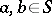(one of these elements may be the empty symbol) and any subsets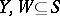, whereis an open subset with compact closure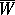and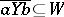or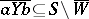, there exist neighbourhoods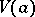and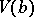ofandsuch that, respectively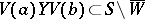. The class of weakly-uniform semi-groups contains all compact semi-groups, discrete semi-groups and locally compact groups. If a locally compact semi-groupis a group, then the mapping of taking the inverse is continuous, i.e.is a topological group. In a locally compact inverse semi-group, this mapping (cf. Regular element) is continuous if and only ifis weakly uniform. In a weakly-uniform semi-group the maximal subgroups are closed. This property need not hold in an arbitrary locally compact semi-group.

An arbitrary compact semi-groupcontains a closed kernel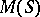(cf. Kernel of a semi-group), which is a completely-simple semi-group. In particular,has idempotents. The structure of compact, completely-simple (completely-simple) semi-groups is described by a theorem analogous to Rees' theorem on discrete completely-simple (completely-simple) semi-groups (cf. Rees semi-group of matrix type). The analogue of Rees' theorem holds for weakly-uniform semi-groups, but not, in general, for locally compact semi-groups .

A semi-groupis called a thread ifcan be linearly ordered in such a way thatbecomes a connected topological semi-group under the order (interval) topology. A semi-groupwith zero 0 and identityis called a standard thread (or-semi-group) ifis a thread and if 0 andare the least and largest elements of. There is a complete description of standard threads . A compact semi-group with identityis called irreducible if it is connected and does not contain a proper connected closed sub-semi-groupfor whichand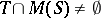. Connected compact semi-groups with identity contain irreducible semi-groups as closed sub-semi-groups. The irreducible semi-groups can be described as follows: An irreducible semi-groupis commutative, the Green equivalence relation(cf. Green equivalence relations) is a closed congruence on, and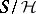is a standard thread.

The "minimal blocks" of a topological semi-group are the closures of its monogenic sub-semi-groups, called monothetic semi-groups. For a compact monothetic semi-groupthe kernelis a compact monothetic group. The compact monothetic semi-groups have been completely described . Weakly-uniform monothetic semi-groups are either compact or discrete. There is an example  of a monothetic locally compact semi-group which is neither discrete nor compact.

A character of a commutative topological semi-group with identity is a non-zero continuous homomorphism into the multiplicative semi-group of complex numbers of modulus. The set of all characters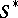forms a commutative topological semi-group with identity with respect to pointwise multiplication (cf. Character of a semi-group) and the compact-open topology. One says that the (Pontryagin) duality theorem holds for a commutative topological semi-group with identity if the canonical homomorphism frominto the semi-group of characters ofis a topological isomorphism "onto" . The duality theorem is true for a commutative compact semi-groupwith identity if and only ifis an inverse semi-group and its sub-semi-group of idempotents forms a totally-disconnected space. Necessary and sufficient conditions have been found  for the duality theorem to hold for a commutative locally compact semi-group. One of the necessary conditions is that the semi-group be weakly uniform.

An important subclass of commutative compact semi-groups are the compact semi-lattices (cf. Idempotents, semi-group of). A compact semi-lattice admits a unique topology, up to a homeomorphism. The description of certain types of topological semi-groups leads to metric semi-groups. A metricon a topological semi-groupis called invariant if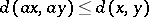and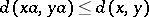for all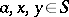. A topological semi-group is called metric if there exists an invariant metric oninducing the topology on. Every compact semi-group is a projective limit of compact metric semi-groups. Every totally-disconnected compact semi-group is a projective limit of finite semi-groups.

Certain generalizations of topological semi-groups have been considered: semi-groups with a non-Hausdorff space, and semi-topological semi-groups, that is, a topological space on which there is defined an associative binary operation such that all left and right inner translations are continuous mappings.

How to Cite This Entry:
Topological semi-group. Encyclopedia of Mathematics. URL: http://encyclopediaofmath.org/index.php?title=Topological_semi-group&oldid=12760
This article was adapted from an original article by B.P. TananaL.N. Shevrin (originator), which appeared in Encyclopedia of Mathematics - ISBN 1402006098. See original article